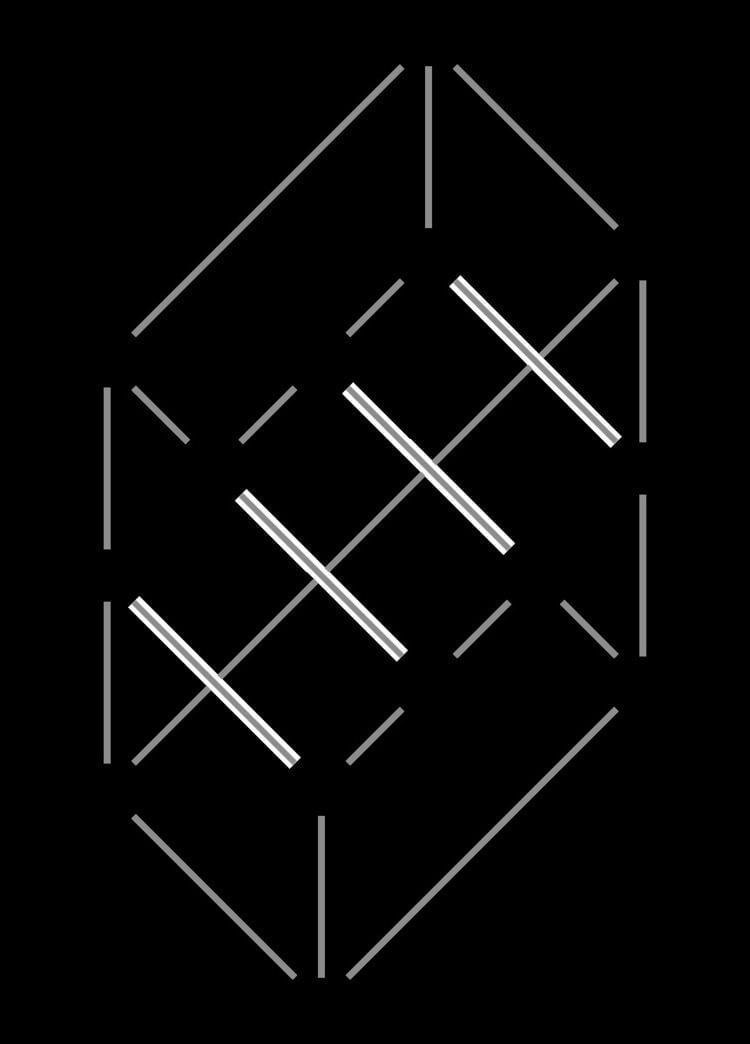# 68 (number)

Updated on
 68  69 →Ordinal  68th(sixty-eighth)Divisors  1, 2, 4, 17, 34, 68 Cardinal  sixty-eightFactorization  2× 17Roman numeral  LXVIII68 (sixty-eight) is the natural number following 67 and preceding 69. It is an even number.

## In mathematics

68 is a Perrin number.

It is the largest known number to be the sum of two primes in exactly two different ways: 68 = 7 + 61 = 31 + 37. All higher even numbers that have been checked are the sum of three or more pairs of primes; the conjecture that 68 is the largest number with this property is closely related to the Goldbach conjecture and like it remains unproven.

Because of the factorization of 68 as 22 × (222 + 1), a 68-sided regular polygon may be constructed with compass and straightedge.

There are exactly 68 10-bit binary numbers in which each bit has an adjacent bit with the same value, exactly 68 combinatorially distinct triangulations of a given triangle with four points interior to it, and exactly 68 intervals in the Tamari lattice describing the ways of parenthesizing five items. The largest graceful graph on 13 nodes has exactly 68 edges. There are 68 different undirected graphs with six edges and no isolated nodes, 68 different minimally 2-connected graphs on seven unlabeled nodes, 68 different degree sequences of four-node connected graphs, and 68 matroids on four labeled elements.

Størmer's theorem proves that, for every number p, there are a finite number of pairs of consecutive numbers that are both p-smooth (having no prime factor larger than p). For p = 13 this finite number is exactly 68. On an infinite chessboard, there are 68 squares three knight's moves away from any cell.

As a decimal number, 68 is the last two-digit number to appear in the digits of pi. It is a happy number, meaning that repeatedly summing the squares of its digits eventually leads to 1:

68 → 62 + 82 = 100 → 12 + 02 + 02 = 1.

## Other uses

• 68 is the atomic number of erbium, a lanthanide
• In the restaurant industry, 68 may be used as a code meaning "put back on the menu", being the opposite of 86 which means "remove from the menu".
• 68 may also be used as slang for oral sex, based on a play on words involving the number 69.
• 68 (number) Wikipedia